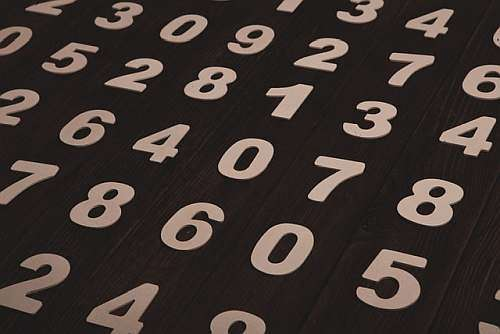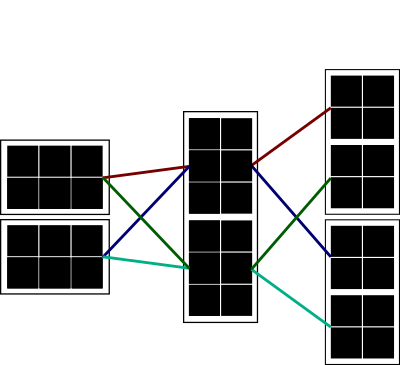# System of Equation Calculator Using Matrices

Instructions: Use this calculator to solve a system of equations you provide by using the inverse of a matrix, showing all the steps. First, click on one of the buttons below to specify the dimension of the system (number of equations and variables). For example, "2x2" means "2 equations and 2 variables"

Then, fill out the coefficients associated to all the variables and the right hand size, for each of the equations. If a variable is not present in one specific equation, type "0" or leave it empty.

x   +   y   +   z   =
x   +   y   +   z   =
x   +   y   +   z   =

## Solving a System of Equation using Matrices

Solving systems of linear equations can be easily one of the most practical skills you will ever learn in Algebra, or even Math overall.

The reason for this is that countless real life applications that are really useful turn out to be solved using systems of linear equations.

There are many methodologies to solve systems, which usually use different approaches. One common approach is the matrix approach, which consists in first converting the system of equation into its matrix form.## How do you solve a system of equations using matrices?

Step 1: Convert the linear equations into matrix from, where you identify $$A$$ (the matrix of coefficients that multiply the corresponding) variables and $$b$$ (the vector of right hand side coefficients).

Step 2: Compute the inverse of the matrix $$A$$, which we call $$A^{-1}$$.

Step 3: The solution of the system is found to be $$x = A^{-1} b$$. In order words, you multiply the inverse of $$A$$ by $$b$$ in order to get the vector with solutions.

Notice that this seems quite simple, but there are lots of computations involved to find the inverse $$A^{-1}$$, particularly if the size of the matrix is large. For a 4x4 and above it can get quite lengthy.

### So, how can you solve systems on a calculator?

The details vary specifically, depending on each calculator. Each machine will have its was and format to input a system. In the case of our calculator, you a get a clear visual panorama of the coefficients you need to fill out in order to specify the system. After that, the calculator will show you all the relevant steps.

### What is consistency of a system of linear equations

Consistency means that the equation do not lead to something that is impossible, like "2 = 3". Typically, before attempting to solve a system, in the case that you have the same number of equations and variables, you first compute the determinant of the matrix.

If the determinant is different from zero, then you can proceed safely with the calculation of the inverse, and you are guaranteed that the system does not have any inconsistency.

### What to do if the matrix is not squared: Gauss elimination

This method of solving a system by computing the inverse of the matrix of coefficients A and multiply it by b only works when the number of variables is the same as the number of equations. If that is not the case, if would be appropriate to use Gauss elimination.### Example

Consider the following system of equations:

\begin{aligned} 2 x&\, + \, & y&\, + \, &2 z & \, = \,1\\ x&\, + \, & y&\, + \, & z & \, = \,2\\ x&\, + \, & y&\, + \, &2 z & \, = \,3 \end{aligned}

Solve the above system using matrices.

Solution: A $$3 \times 3$$ system of linear equations has been provided and we need to solve this system using matrices.

### Step 1: Find the corresponding Matrix Structure

The first step consists of finding the corresponding matrix $$A$$ and vector $$b$$ that allow the system to be written as $$A x = b$$.

In this case, and based on the coefficients of the equations provided, we get that

$A = \begin{bmatrix} \displaystyle 2&\displaystyle 1&\displaystyle 2\\[0.6em]\displaystyle 1&\displaystyle 1&\displaystyle 1\\[0.6em]\displaystyle 1&\displaystyle 1&\displaystyle 2 \end{bmatrix}$

and

$b = \begin{bmatrix} \displaystyle 1\\[0.6em]\displaystyle 2\\[0.6em]\displaystyle 3 \end{bmatrix}$

### Step 2: Compute the Determinant of the Matrix

Now, we need to compute the determinant of $$A$$ in order to know whether or not we can compute the inverse of the matrix $$A$$:

Using the sub-determinant formula we get:

$\begin{vmatrix} \displaystyle 2&\displaystyle 1&\displaystyle 2\\[0.6em]\displaystyle 1&\displaystyle 1&\displaystyle 1\\[0.6em]\displaystyle 1&\displaystyle 1&\displaystyle 2 \end{vmatrix} = 2 \cdot \left( 1 \cdot \left( 2 \right) - 1 \cdot \left(1 \right) \right) - 1 \cdot \left( 1 \cdot \left( 2 \right) - 1 \cdot \left(1 \right) \right) + 2 \cdot \left( 1 \cdot \left( 1 \right) - 1 \cdot \left(1 \right) \right)$ $= 2 \cdot \left( 1 \right) - 1 \cdot \left( 1 \right) + 2 \cdot \left( 0 \right) = 1$

Since $$\det(A) = \displaystyle 1 \ne 0$$, we conclude that the matrix is invertible, and we can continue with the calculation of the inverse.

### Step 3: Computing the Inverse

Now we compute the minors matrix. We have that, by definition, the minors matrix $$M$$ is defined by the formula

$M_{ij} = \det A^{i,j}$

where in this case $$A^{i,j}$$ is the matrix $$A$$ after deleting row $$i$$ and column $$j$$.

Therefore, and based on the matrix $$A$$ provided we get the following coefficients of the minors matrix:

For $$A^{ 1, 1}$$:

$M_{ 1 1} = \det A^{ 1 1} = \begin{vmatrix} \displaystyle 1&\displaystyle 1\\[0.6em]\displaystyle 1&\displaystyle 2 \end{vmatrix} = 1 \cdot \left( 2 \right) - 1 \cdot \left(1 \right) = 1$

For $$A^{ 1, 2}$$:

$M_{ 1 2} = \det A^{ 1 2} = \begin{vmatrix} \displaystyle 1&\displaystyle 1\\[0.6em]\displaystyle 1&\displaystyle 2 \end{vmatrix} = 1 \cdot \left( 2 \right) - 1 \cdot \left(1 \right) = 1$

For $$A^{ 1, 3}$$:

$M_{ 1 3} = \det A^{ 1 3} = \begin{vmatrix} \displaystyle 1&\displaystyle 1\\[0.6em]\displaystyle 1&\displaystyle 1 \end{vmatrix} = 1 \cdot \left( 1 \right) - 1 \cdot \left(1 \right) = 0$

For $$A^{ 2, 1}$$:

$M_{ 2 1} = \det A^{ 2 1} = \begin{vmatrix} \displaystyle 1&\displaystyle 2\\[0.6em]\displaystyle 1&\displaystyle 2 \end{vmatrix} = 1 \cdot \left( 2 \right) - 1 \cdot \left(2 \right) = 0$

For $$A^{ 2, 2}$$:

$M_{ 2 2} = \det A^{ 2 2} = \begin{vmatrix} \displaystyle 2&\displaystyle 2\\[0.6em]\displaystyle 1&\displaystyle 2 \end{vmatrix} = 2 \cdot \left( 2 \right) - 1 \cdot \left(2 \right) = 2$

For $$A^{ 2, 3}$$:

$M_{ 2 3} = \det A^{ 2 3} = \begin{vmatrix} \displaystyle 2&\displaystyle 1\\[0.6em]\displaystyle 1&\displaystyle 1 \end{vmatrix} = 2 \cdot \left( 1 \right) - 1 \cdot \left(1 \right) = 1$

For $$A^{ 3, 1}$$:

$M_{ 3 1} = \det A^{ 3 1} = \begin{vmatrix} \displaystyle 1&\displaystyle 2\\[0.6em]\displaystyle 1&\displaystyle 1 \end{vmatrix} = 1 \cdot \left( 1 \right) - 1 \cdot \left(2 \right) = -1$

For $$A^{ 3, 2}$$:

$M_{ 3 2} = \det A^{ 3 2} = \begin{vmatrix} \displaystyle 2&\displaystyle 2\\[0.6em]\displaystyle 1&\displaystyle 1 \end{vmatrix} = 2 \cdot \left( 1 \right) - 1 \cdot \left(2 \right) = 0$

For $$A^{ 3, 3}$$:

$M_{ 3 3} = \det A^{ 3 3} = \begin{vmatrix} \displaystyle 2&\displaystyle 1\\[0.6em]\displaystyle 1&\displaystyle 1 \end{vmatrix} = 2 \cdot \left( 1 \right) - 1 \cdot \left(1 \right) = 1$

Summarizing, the minors matrix is:

$M = \begin{bmatrix} \displaystyle 1&\displaystyle 1&\displaystyle 0\\[0.6em]\displaystyle 0&\displaystyle 2&\displaystyle 1\\[0.6em]\displaystyle -1&\displaystyle 0&\displaystyle 1 \end{bmatrix}$

Now, we can compute the elements of the cofactor matrix $$C$$ using the formula

$C_{ij} = (-1)^{i+j}M_{ij}$

The above formula can be used directly because the minors are known already. We get

$C_{ 1 1} = (-1)^{ 1+1} \cdot 1 = (-1)^{ 2} \cdot 1 = 1$ $C_{ 1 2} = (-1)^{ 1+2} \cdot 1 = (-1)^{ 3} \cdot 1 = -1$ $C_{ 1 3} = (-1)^{ 1+3} \cdot 0 = (-1)^{ 4} \cdot 0 = 0$ $C_{ 2 1} = (-1)^{ 2+1} \cdot 0 = (-1)^{ 3} \cdot 0 = 0$ $C_{ 2 2} = (-1)^{ 2+2} \cdot 2 = (-1)^{ 4} \cdot 2 = -2$ $C_{ 2 3} = (-1)^{ 2+3} \cdot 1 = (-1)^{ 5} \cdot 1 = -1$ $C_{ 3 1} = (-1)^{ 3+1} \left(-1\right)= (-1)^{ 4} \left(-1\right) = 1$ $C_{ 3 2} = (-1)^{ 3+2} \cdot 0 = (-1)^{ 5} \cdot 0 = 0$ $C_{ 3 3} = (-1)^{ 3+3} \cdot 1 = (-1)^{ 6} \cdot 1 = -1$

Hence, the cofactor matrix is:

$C = \begin{bmatrix} \displaystyle 1&\displaystyle -1&\displaystyle 0\\[0.6em]\displaystyle 0&\displaystyle -2&\displaystyle -1\\[0.6em]\displaystyle 1&\displaystyle 0&\displaystyle -1 \end{bmatrix}$

Now, we need just need to transpose the cofactor matrix we have found to compute the adjoint matrix. We get:

$adj(A) = C^T = \begin{bmatrix} \displaystyle 1&\displaystyle -1&\displaystyle 0\\[0.6em]\displaystyle 0&\displaystyle -2&\displaystyle -1\\[0.6em]\displaystyle 1&\displaystyle 0&\displaystyle -1 \end{bmatrix} ^T = \begin{bmatrix} \displaystyle 1&\displaystyle 0&\displaystyle 1\\[0.6em]\displaystyle -1&\displaystyle -2&\displaystyle 0\\[0.6em]\displaystyle 0&\displaystyle -1&\displaystyle -1 \end{bmatrix}$

Finally, we need to multiply every component of the adjoint matrix by $$\displaystyle \frac{1}{\det(A)} = \frac{1}{1} = 1$$, which does not affect the adjoint. So we get:

$A^{-1} = \frac{1}{\det(A)} adj(A) = \frac{1}{1} \begin{bmatrix} \displaystyle 1&\displaystyle 0&\displaystyle 1\\[0.6em]\displaystyle -1&\displaystyle -2&\displaystyle 0\\[0.6em]\displaystyle 0&\displaystyle -1&\displaystyle -1 \end{bmatrix} = \begin{bmatrix} \displaystyle 1&\displaystyle 0&\displaystyle 1\\[0.6em]\displaystyle -1&\displaystyle -2&\displaystyle 0\\[0.6em]\displaystyle 0&\displaystyle -1&\displaystyle -1 \end{bmatrix}$

### Step 4: Computing the Solutions

Now that we know the inverse $$A^{-1}$$, the vector of solutions is computed as:

$x = A^{-1} b = \begin{bmatrix} \displaystyle 1&\displaystyle 0&\displaystyle 1\\[0.6em]\displaystyle -1&\displaystyle -2&\displaystyle 0\\[0.6em]\displaystyle 0&\displaystyle -1&\displaystyle -1 \end{bmatrix} \begin{bmatrix} \displaystyle 1\\[0.6em]\displaystyle 2\\[0.6em]\displaystyle 3 \end{bmatrix} = \begin{bmatrix} \displaystyle 1\cdot 1+0\cdot 2+1\cdot 3\\[0.6em]\displaystyle -1\cdot 1+\left(-2\right)\cdot 2+0\cdot 3\\[0.6em]\displaystyle 0\cdot 1+\left(-1\right)\cdot 2+\left(-1\right)\cdot 3 \end{bmatrix} = \begin{bmatrix} \displaystyle -2\\\\\displaystyle 3\\\\\displaystyle 1 \end{bmatrix}$

Hence, and summarizing, the solution vector is

$\begin{bmatrix} \displaystyle x\\\\\displaystyle y\\\\\displaystyle z \end{bmatrix} = \begin{bmatrix} \displaystyle -2\\\\\displaystyle 3\\\\\displaystyle 1 \end{bmatrix}$

which concludes the calculation of the solutions for the given linear system.